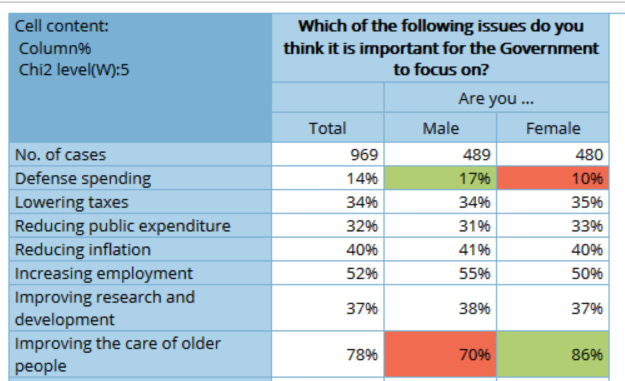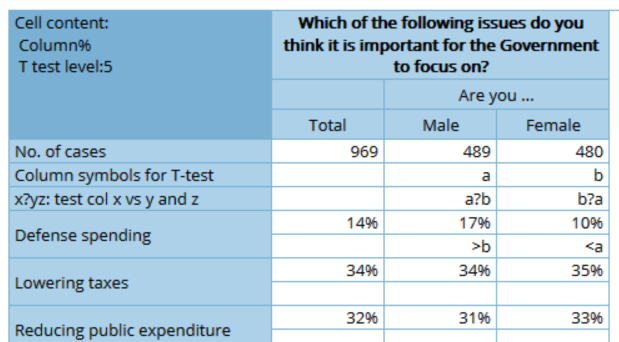# How do I enable statistical tests in cross tables?

Superdig has included significance testing as basic functionality. By this you will be able to see which categories differ from total (Chi-2) or from each other (t-test) in a simple way.

By default all tables are having Chi2 (“Chi-square”)-significance testing included. You may modify or turn these off, either by selecting the table(s) or changing default values

To specify significance testing:

1. Specify whether you want to run Chi-2 testing, t-testing or both
2. Specify at which level you want to run the tests at.
3. For Chi2-tests, the following apply:
1. 0 (Zero) the test level is determined by the table filter number of cases as follows: 10% when cases = 1-300, 5% (95% confidence inetrval) when cases = 301-1000, 2,5% when cases = 1001-4000, 0,5% when cases = 4001-20000 0,1% when cases = 20001 or more
2. By specifying a number “N”, the test level will override the automatic level. The value is restricted to values ranging between 0 and 50.
3. The results of the significance test will be visualized as colored cells in the table
1. Green cells respectively red cells indicating a significantly higher (lower) level than the “Total” column
2. The level at which the table has been run will be shown in the documentation cell in the upper left corner of the table
4.  For t-tests, the following apply
1. 0 (Zero) the test level is determined by the table filter number of cases as follows: 10% (90% confidence interval) when cases = 1-200, 5% when cases = 201-400, 2% when cases = 401-800, 1% when cases = 801-1600, 0,5% when cases = 1601-3200, 0,2% when cases = 3201-6400, 0,1% when cases = 6401 or more
2. By specifying a number “N”, the test level will override the automatic level. The value is restricted to values ranging between 0 and 50.
3. The results of the significance test will be visualized as follows:
1. A column-naming shown at the top of each column (“Column symbols for T-test”) This will be a letter from a-z
2. A documentation which columns that are tested towards each other (a?b means that column a has been tested towards column b)
3. A greater than / less than sign specifying in which the cell significantly higher / lower is (If a table cell in column "a" says “>b” means that the figure in column a is greater than the corresponding figure in column b)

Example showing Chi2 test at 5%Example showing T-test at 5%